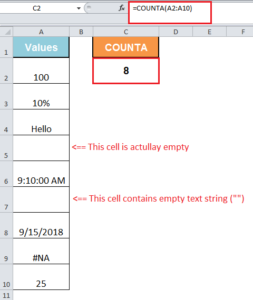Get instant live expert help with Excel or Google Sheets“My Excelchat expert helped me in less than 20 minutes, saving me what would have been 5 hours of work!”

#### Post your problem and you’ll get expert help in seconds.

Your message must be at least 40 characters
Our professional experts are available now. Your privacy is guaranteed.

# Learn How to Use the COUNTA Function in Excel

When you enter data in Excel, you sometimes do it with a lot of cells left blank to fill them at a later stage when data is available. When you want to count the number of entries you made in a cell range, then you need a specific function in Excel to count only cells that are filled or Non-Blank. For this purpose, you need to use a special built-in function that is called the COUNTA function.

## Using the COUNTA function in Excel

COUNTA Excel function returns the count of cells that are not empty or Non-Blank. It returns a number representing a count of values. It counts cells that contain numbers, text, date values, time values, logical values (TRUE and FALSE), error values, and empty text (“”), but it does not count empty cells.

The syntax of COUNTA Excel function is;

`=COUNTA (value1, [value2], ...)`

It supports up to 255 value arguments. A value argument could be a hardcoded value, cell reference or a range.

In this article, you will learn how to use the COUNTA in Excel. The syntax of this function is very easy to follow. If you have all the data in one range, then you need to enter only one range in COUNTA function to count nonempty cells. Like, if you have a range A1:A100 from which you want to count cells containing values, so you will use this range as follows;

`=COUNTA(A1:A100)`

But if you have data in various ranges, and you want to count values from multiple ranges in one go, then you will enter each range as a value argument in COUNTA Excel function, such as;

`=COUNTA(B1:B100,J2:K50,M1:N100)`

COUNTA function may count those cells that are visually empty, but actually, they are not as these cells may contain empty text (“”) or space and COUNTA function consider such entries as value. So it only ignores those cells that are actually empty.

Suppose you have various types of values in a range of cells A2:A10 and you want to count cells containing values, excluding only those cells that you are actually empty, not visually empty.So visually there are 7 cells that have values in range A2:A10, but COUNTA function returns the result as 8 cells that are non-empty. This is because of cell A7 that contains empty text string (“”), and it is counted in COUNTA function as a value.

Still need some help with Excel formatting or have other questions about Excel? Connect with a live Excel expert here for some 1 on 1 help. Your first session is always free.

### Did this post not answer your question? Get a solution from connecting with the expert.Another blog reader asked this question today on Excelchat:
Related blogs
Solution examplesUse the Vlookup Function to complete the "employee" column of table 2. Use "job Id" from table 2 as your lookup_value(s) and table 1 as your reference.
Solved by C. H. in 16 minsIf a cell in another sheet is populated I need a vlookup done. If the cell is not populated I need the cell to return blank.
Solved by T. D. in 60 minsI am trying to make a chart that turns a week range red if nothing is entered in the range. If something is entered then I would like it to turn green. Please Help
Solved by E. U. in 43 minsI need a check box to show/hide an answer of an if function
Solved by Z. U. in 23 minsI need a formula to compare the data in two columns and then export the mismatched data in the 3rd column
Solved by S. Q. in 20 mins## Subscribe to Excelchat.coAnother blog reader asked this question today on Excelchat: